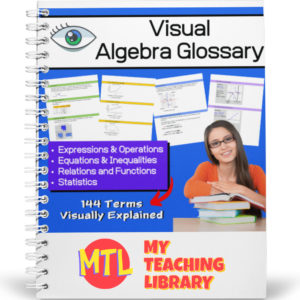Showing all 7 results

• Sale!\$7.00 \$5.50

This consumer math bundle will teach students about both simple and compound interest and give them the practice they need to help them master solving word problems!

Includes:

•\$4.00

This consumer math resource has been designed to help teach students and give them the practice they need to master the concepts taught.

The first two pages will explain interest (simple and compound) and give students the formulas they will need to solve the word problems. There are 60 word problems in all. 3 sets of 10 simple interest problems and 3 sets of 10 compound interest problems. Answer keys are provided.

•\$3.50

Give students the practice they need when learning to solve one-step, one-variable equations! This resource provides 7 different worksheets, each with 25 problems for a total of 175 problems. All equations in this set of worksheets use only the properties of addition and subtraction.

•\$15.00

Give your Algebra I and Algebra II students a tool they can really use! This Visual Algebra Glossary will be an invaluable resource giving them explanations, definitions and examples of 144 terms. Easy to follow and understand, students will turn to this resource while learning new concepts, completing work and studying for exams.

There are four color coded sections, each with an term index:

• – Expressions & Operations
• – Equations & Inequalities
• – Relations & Functions
• – Statistics

73 pages

•\$3.00

This resource is designed to give students the practice the need to become proficient at multiplying decimal numbers. There are three levels, 15 worksheets (20 problems each) and answer keys. That’s 300 problems! Use as practice sheets, homework, or for quizzes and tests.

Also included: Visual poster to help students with ‘where to place’ the decimal point in their answers.

3 Levels:

• Level 1 (5 worksheets)
Tenths multiplied by tenths
• Level 2 (5 worksheets)
Tenths multiplied by hundreds and/or by thousandths
Hundredths multiplied by hundredths and/or by thousandths
• Level 3 (5 worksheets)
Mixed from tenths/hundredths/thousandths multiplied by tenths/hundredths/thousandths
•\$4.00

This 123 page math resource will help students who are learning (or struggling to understand) algebra better by giving detailed explanations of related terminology and concepts. This book as been categorized as curriculum because of the detailed explanations of the topics. It however, is for instructional use only and does not include student problems.

(See description below for covered topics)

•\$9.99

A step by step Geometry curriculum to teach students with ease. Students will learn about angles, lines, triangles, polygons,quadrilaterals, prisms, coordinate geometry, slope and more!